# KSEEB SSLC Class 10 Maths Solutions Chapter 5 Areas Related to Circles Ex 5.3

In this chapter, we provide KSEEB SSLC Class 10 Maths Solutions Chapter 5 Areas Related to Circles Ex 5.3 for English medium students, Which will very helpful for every student in their exams. Students can download the latest KSEEB SSLC Class 10 Maths Solutions Chapter 5 Areas Related to Circles Ex 5.3 pdf, free KSEEB SSLC Class 10 Maths Solutions Chapter 5 Areas Related to Circles Ex 5.3 book pdf download. Now you will get step by step solution to each question.

### Karnataka State Syllabus Class 10 Maths SolutionsChapter 5 Areas Related to Circles Ex 5.3

{Unless stated otherwise, use π=227}

Question 1.
Find the area of the shaded region in the given figure, if PQ = 24 cm, PR = 7 cm and O is the centre of the circle.
Solution: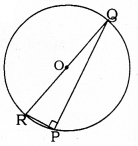PQ = 24 cm, PR = 7 cm,
‘O’ is the centre of circle.
Angle in semicircle,
∠RPQ = 90°
In ⊥∆RPQ, ∠P = 90°
RQ = RP2 + PQ2
= (7)2 + (24)2
= 49 + 576
RQ2 = 625
∴ RQ = 25 cm.
Diameter, RQ = 25 cm.
∴ Radius, OR = OQ = 252 cm.
= Area of semicircle – Area of ∆RPQ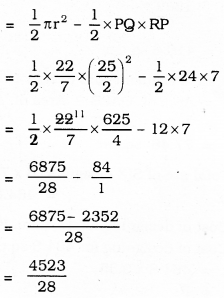= 161.3 sq.cm.

Question 2.
Find the area of the shaded region in the given figure, if radii of the two concentric circles with centre O are 7 cm and 14 cm respectively and ∠AOC = 40°.
Solution: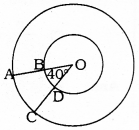Radius of small circle, r = 7 cm
Radius of big circle, R = 14 cm
∠AOC = θ = 40°
Area of shaded part, ABCD =?
Area of ABCD
= Area of OAC – Area of OBD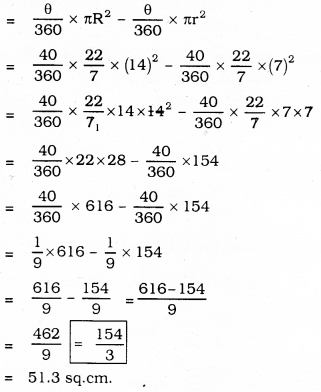Question 3.
Find the area of the shaded region in the given figure, if ABCD is a square of side 14 cm and APD and BPC are semicircles.
Solution: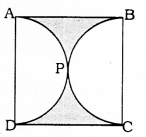Each side of square ABCD = 14 cm.
APD, APC are semicircles.
Area of the shaded region = ?
i) Total area of square
= (Side)2
= (14)2
= 196 sq.cm.

ii) Each radius, r of semicircle
r = 142 =7cm
Area of two semicircles :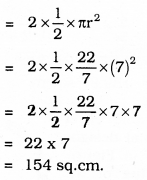= Area of square – Area of two semicircles
= 196 – 154
= 42 sq. cm

Question 4.
Find the area of the shaded region in the given figure, where a circular arc of radius 6 cm has been drawn with vertex O of an equilateral triangle OAB of side 12 cm as centre.
Solution: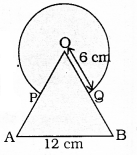OAB is an equilateral Triangle with side 12 Circle with radius of 6
Each angle of an equilateral triangle is 60°
Angle at the centre in segment, θ = 60°
Area of Major segment + Area of an equilateral triangle OAB =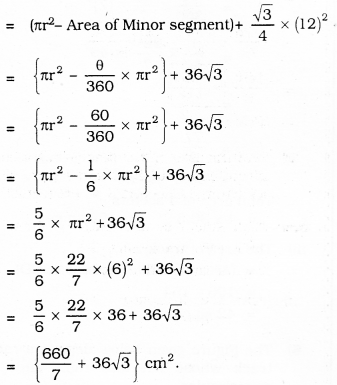= 94.28 + 36 × 1.73
= 94.28 + 62.28
= 156.56 sq. cm.

Question 5.
From each corner of a square of side 4 cm a quadrant of a circle of radius 1 cm is cut and also a circle of diameter 2 cm is cut as shown in the given figure. Find the area of the remaining portion of the square.
Solution:
i) Each side of square ABCD = 4 cm.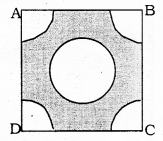∴ Area of Square:
= (Side)2
= (4)2
= 16 sq.cm.

(ii) Area of circle in the corner A is 1 cm.
θ = 90°
∴ Area of quadrant of circle: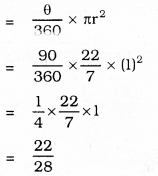= 0.78 sq.cm.

iii) Radius of circle having diameter 2 cm, r = 1 cm.
∴ Area of circle: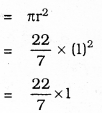= 3.14 sq. cm.

∴ Area of remaining part of Square :
= Area of Square – Area of 4 quadrants – Area of Circle.
= 16 – 4 × 0.78 – 3.14
= 16 – 3.12 – 3.14
= 16 – 6.26
= 9.74 sq.cm.

Question 6.
In a circular table cover of radius 32 cm, a design is formed leaving an equilateral triangle ABC in the middle as shown in the given figure. Find the area of the design.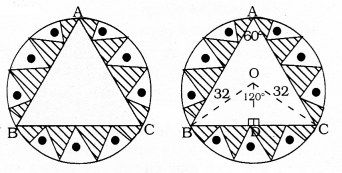Solution:
Radius of the circle r = 32 cm.
∴ Area of the circle = πr2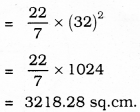ii) ∠BAC = 60° ∴ ∠BOC = 120°
(∵ Angle at the centre is double than the angle in the circumference).
Now, in ∆OBC, BC ⊥ OD,
∠BOD = ∠COD = 60°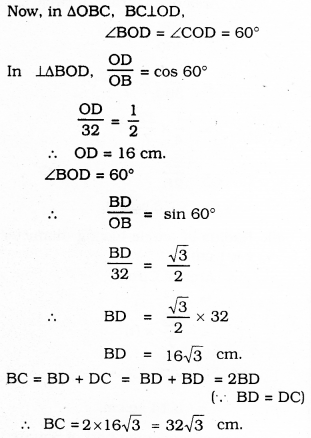∴ ∆ABC is an equilateral triangle.
Each side of this triangle,
a= 2 × 163–√=323–√cm
Area of equilateral triangle, ∆ABC :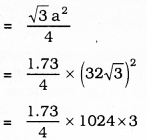= 1328.64 sq.cm,

iii) Area of shaded region :
= Area of circle – Area of an equilateral ∆le
= 3218.28 – 1328.64
= 1889.6 sq.cm.

Question 7.
In the Figure given below, ABCD is a square of side 14 cm. With centres A, B, C and D, four circles are drawn such that each circle touch externally two of the remaining three circles. Find the area of the shaded region.
Solution: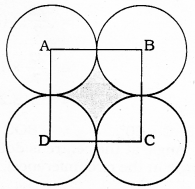i) Each side of square ABCD =14 cm.
∴ Area of square ABCD
= (Side)2
= (14)2
= 196 sq.cm.

ii) Measure of radii of 4 circles, r = 142= = 7 cm.
∵ Distance between tangents which touches circles externally,
d = R + r = 7 + 7 = 14 cm.
Area of segment with centre, A = ?
r = 7 cm, θ = 90°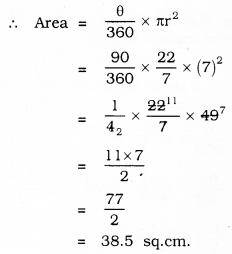∴ Total area of 4 sectors
= 4 × 38.5 = 154 sq.cm.

iii) The Area of track :
= (Area of GHIJ – Area of ABCD)
= 196 – 154
= 42 sq.cm.

Question 8.
The Figure given below depicts a racing track whose left and right ends are semicircular.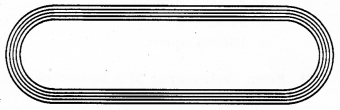The distance between the two inner parallel line segments is 60 m and they are each 106 m long. If the track is 10 m wide, find:
i) The distance around the track along its inner edge,
ii) The area of the track.
Solution: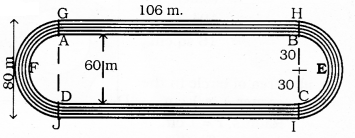The distance around the track along its inner edge:
= AB + Arc BEC + CD + Area of Arc DFA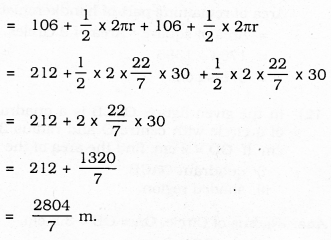The Area of Track:
= (Area of GHIJ – Area of ABCD) + (Area of semicircle HKI – Area of semicircle BEC) + (Area of semicircle GIJ – Area of semicircle AFD)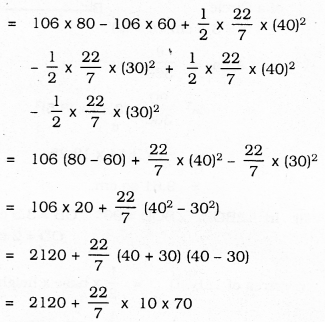= 2120 + 2200
= 4320 sq.m.
∴ Total area of Track = 4320 sq.m.

Question 9.
In the figure given below, AB and CD are two diameters of a circle (with centre O) perpendicular to each other and OD is the diameter of the smaller circle. If OA = 7 cm, find the area of the shaded region.
Solution: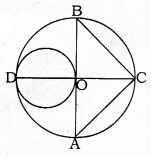i) Radius of Big circle is the diameter of a small circle.
= 72 = 3.5 cm
∴ Area of smalle circle = πr2
= 227 × (3.5)2
= 227 × 12.25
= 3.14 × 12.25
= 38.47 sq.cm.

ii) Area of segment OCB = ?
Radius, r = 7 cm, θ = 90°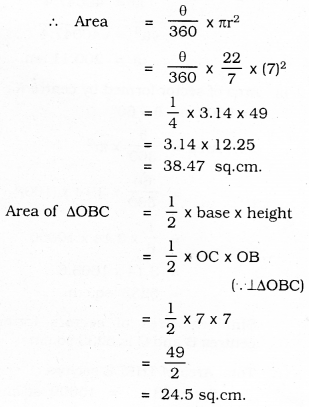∴ Area of sector OCB – Area of ∆OBC
= 38.47 – 24.50
= 13.97 sq.cm.
Similarly, Area of sector OAC – Area of ∆OAC
= 38.47 – 24.50
= 13.97 sq.cm.
∴ Total area of shaded region :
= Area of small circle + Area of Minor segment OBC + Area of Minor segment OAC
= 38.47 + 13.97 + 13.97
= 66.41 sq.cm.

Question 10.
The area of an equilateral triangle ABC is 17320.5 cm2. With each vertex of the triangle as centre, a circle is drawn with radius equal to half the length of the side of the triangle (see the Figure). Find the area of the shaded region.
(Use π = 314 and 3–√ = 1.73205).
Solution: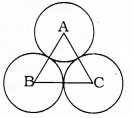i) Area of an equilateral ∆ABC :
= 17320.5 cm2
3√a24 = 17320.5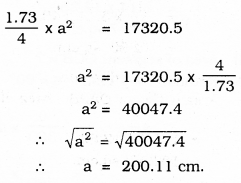ii) Area formed by centre A:
r = 100 cm, θ = 60°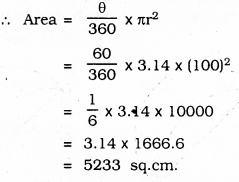Similarly Area of sectors formed by centres B and C is 5233 sq.cm.
∴ Total Area of THREE sectors :
= 3 × 5233 = 15699 sq.cm.
∴ Area of the shaded region:
= Area of an equilateral Triangle – Area of three sectors.
= 17320.5 – 15699
= 1621.5 sq. cm.

Question 11.
On a square handkerchief, nine circular designs each of radius 7 cm are made (see the Figure). Find the area of the remaining portion of the handkerchief.
Solution: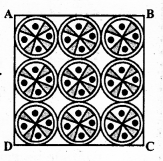i) Total area of 9 circular designs each of radius 7 cm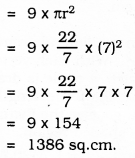ii) All the circles touches externally.
∴ Sum of the diameter of 3 circles in first Row =
14 + 14 + 14 = 42 cm.
∴ Length of each side of square ABCD,
a = 42 cm.
∴ Area of square ABCD = a2
= (42)2
= 1764 sq.cm.
Area of remaining part of handkerchief:
= Area of a square – Area of 9 circles.
= 1764 – 1386
= 378 sq.cm.

Question 12.
In the given figure, OACB is a quadrant of a circle with centre O and radius 3.5 cm If OD = 2 cm, find the area of the
Solution:
Radius of Circle, OA = OB = 3.5 cm.
OD = 2 cm.
i) Area of a quadrant of a circle
ii) Area of the shaded region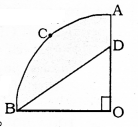i) Area of quadrant (OACB) of a Circle
r = 3.5 cm, θ = 90°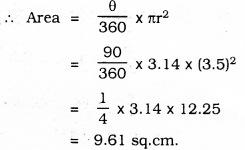ii) In ⊥∆BOD, ∠BOD = 90° OB = 3.5 cm; OD = 2 cm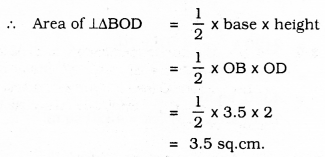= Area of quadrant OACB – Area of ABOD
= 9.61 – 3.5
= 6.11 sq.cm.

Question 13.
In Figure (i) given below, a square OABC is inscribed in a quadrant OPBQ. If OA = 20 cm, find the area of the shaded region. (Use π = 3.14)
Solution: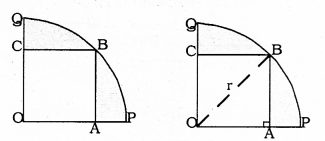i) Each side of square OABC = 20 cm.
∴ OA = 20 cm, AB = 20 cm.
∴ Area of square PABC = (Side)2
= (20)2
= 400 sq.cm.

ii) Diagonal OB is drawn in OABC square.
In ⊥∆OAB, OA = 20 cm.
AB = 20 cm.
OB =?
OB2 = OA2 + AB2
= (20)2 + (20)2
= 400 + 400
= 800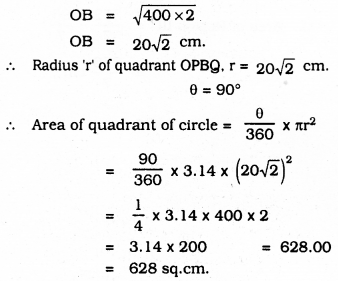∴ Area of shaded region = 628 – 400 = 228 cm2.

Question 14.
AB and CD are respectively arcs of two concentric circles of radii 21 cm and 7 cm and centre O (see Figure given below). If ∠AOB = 30°, find the area of the shaded region.
Solution:
i) Area of segment OAPB = ?
r = 21 cm, θ = 30°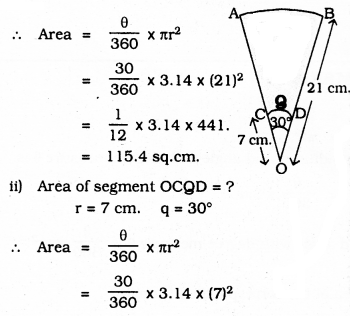= 112 × 3.14 × 49
= 12.82 sq.cm.
∴ Area of the shaded region = Area of Sector OAPB – Area of segment OCQD
= 115.4 – 12.82
= 102.58 sq.cm.

Question 15.
In the given figure (i), ABC is a quadrant of a circle of radius 14 cm and a semicircle is drawn with BC as diameter. Find the area of the shaded region.
Solution: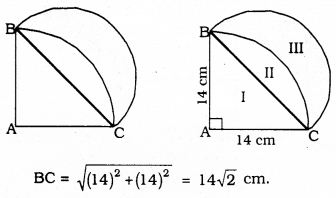i) Area of Part II :
Area of Segment ABC – Area of ∆ABC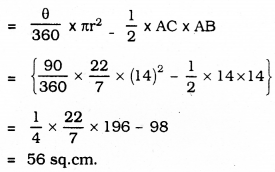= 56 sq.cm.

ii) Area of shaded region Part III :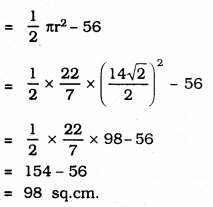Question 16.
Calculate the area of the designed region in the given figure common between the two quadrants of circles of radius 8 cm each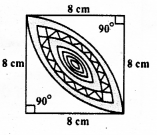Solution: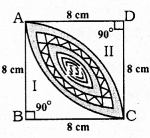i) Area of Square, ABCD = a2 = (8)2 = 64 cm2.
ii) Sum of Areas of Part II and Part III = Area of the segment with centre D and radius of 8 cm.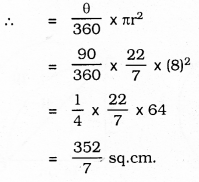iii) Area of Part I = (Sum of Part I, II and III) – (Sum of the area of Part II, III)
= Area of Square ABCD – (Sum of the area of part II and III)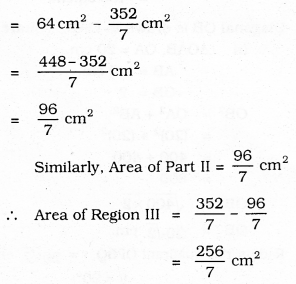All Chapter KSEEB Solutions For Class 10 Maths

—————————————————————————–

All Subject KSEEB Solutions For Class 10

*************************************************

I think you got complete solutions for this chapter. If You have any queries regarding this chapter, please comment on the below section our subject teacher will answer you. We tried our best to give complete solutions so you got good marks in your exam.

If these solutions have helped you, you can also share kseebsolutionsfor.com to your friends.

Best of Luck!!